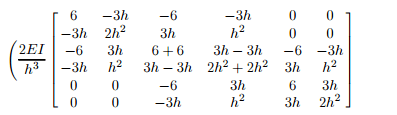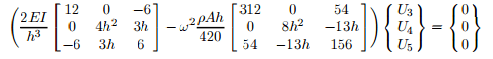# 5482

Determine the smallest natural frequency of a beam with clamped ends, and of constant cross-sectional area A, moment of inertia I, and length L. Use the symmetry and two Euler—Bernoulli beam elements in the half beam

sol\$

Note that the beam problem is a hyperbolic equation, hence the eigenvalue is the square of the natural frequency of flexural vibration, ω. For a mesh of two Euler—Bernoulli elements in a half beam (i.e., h = L/4), the assembled equations are given by

### Save your time - order a paper!

Get your paper written from scratch within the tight deadline. Our service is a reliable solution to all your troubles. Place an order on any task and we will take care of it. You won’t have to worry about the quality and deadlines

Order Paper NowThe boundary conditions are: U1 = U2 = U6 = 0 and Q2/ 3 = 0. The condensed equations are given byThe determinant of the coefficient matrix yields a cubic polynomial in ω2. Note that by considering the half beam we restricted the natural frequencies to those of symmetric modes. The antisymmetric modes (only) can be obtained by using U5 = 0 instead of U6 = 0.